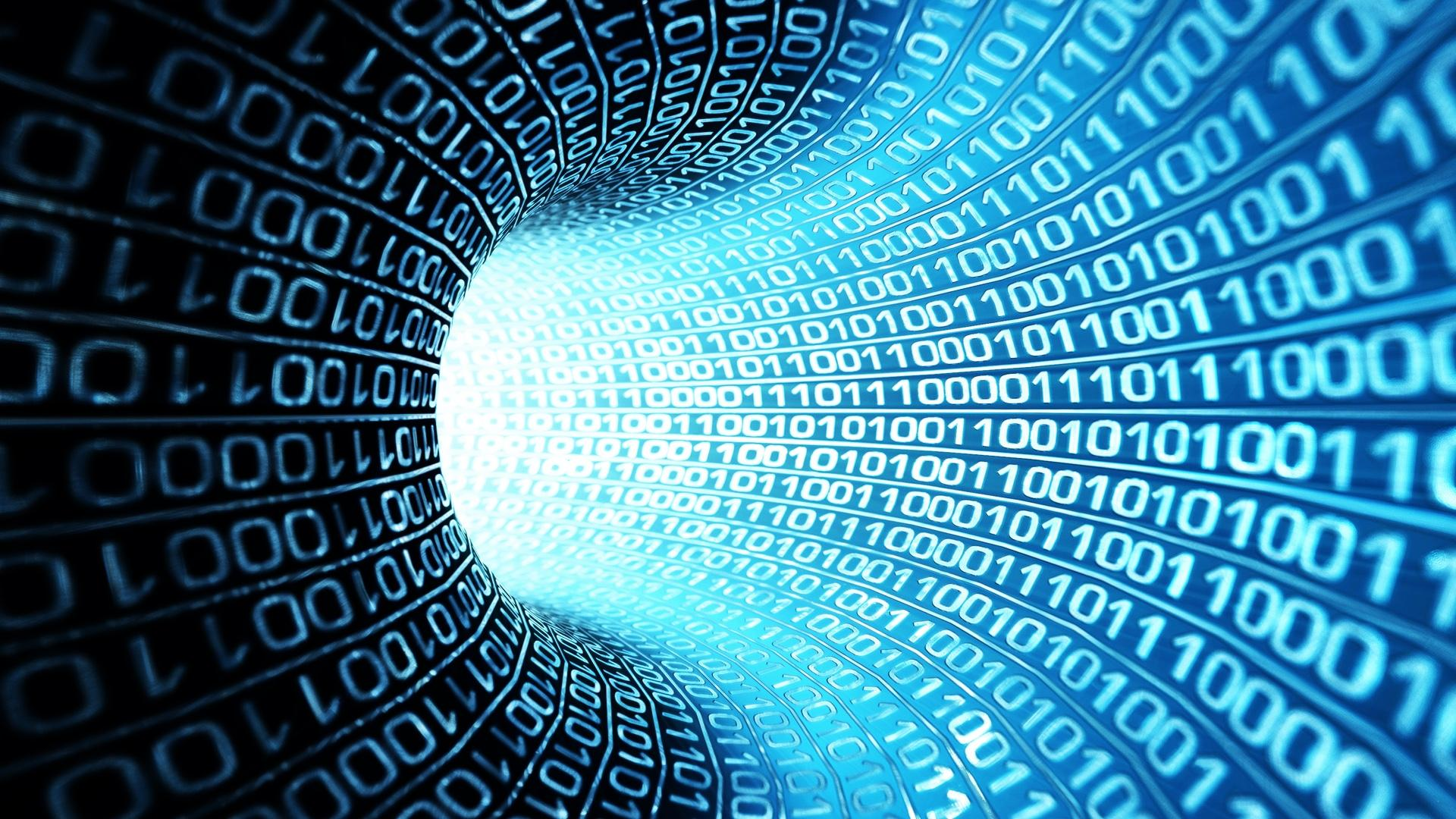### Bits and Bytes of ComputerWe all use computer and came across terms like bits,bytes,KB,MB,GB in terms of storage…..what are these and where do they come from??

Starting from the Number Forms :

Binary Form (only 2 digits) — 0,1

Octal Form (only 8 digits) — 0 to 7

Decimal Form (only 10 digits) — 0 to 9

HexaDecimal Form ( 10 digits and 6 characters) — 0 to 9,A=10,B=11,C=12,D=13,E=14,F=15

So if you see a number like 5de73 then its of hexadecimal form and each form can be converted to other forms.

each of these digits when converted to its consequent binary form, each binary digit occupy 1-bit in computer and with the increase of each bit or character you increase a bit, so here is the overview of storage

Bit = 1 Binary Digit

Nibble = 4 Bit

Byte = 8 Bit

Kilobyte = 1024 Byte

Megabyte = 1024 Kilobyte

Gigabyte = 1024 Megabyte

Terabyte = 1024 Gigabyte

Petabyte = 1024 Terabyte

Exabyte = 1024 Petabyte

Zettabyte = 1024 Exabyte

Yottabyte = 1024 Zettabyte

Brontobyte = 1024 Yottabyte

Geopbyte = 1024 Brontobyte

Saganbyte = 1024 Geopbyte

Pijabyte = 1024 Saganbyte

Alphabyte = 1024 Pijabyte

Kryatbyte = 1024 Alphabyte

Amosbyte = 1024 Kryatbyte

Pectrolbyte = 1024 Amosbyte

Bolgerbyte = 1024 Pectrolbyte

Sambobyte = 1024 Bolgerbyte

Quesabyte = 1024 Sambobyte

Kinsabyte = 1024 Quesabyte

Rutherbyte = 1024 Kinsabyte

Dubnibyte = 1024 Rutherbyte

Seaborgbyte = 1024 Dubnibyte

Bohrbyte = 1024 Seaborgbyte

Hassiubyte = 1024 Bohrbyte

Meitnerbyte = 1024 Hassiubyte